# Crossing Swords

## OBJECTIVE: To learn how to compare fractions

In a previous lesson, you learned how to SIMPLIFY FRACTIONS. Cutting Down to Size: https://www.geogebra.org/m/y2v77wpx In this lesson, you're going to learn how to COMPARE FRACTIONS. There are many approaches to comparing fractions. Here, we just focus on the CRISS-CROSS METHOD. While it is not absolutely necessary to reduce fractions to lowest terms before comparing them, it is preferable to do so in order to avoid having to work with large numbers. Here are the steps: Step 1: Write down the two fractions side by side. Step 2: Cross-multiply the denominator of the first fraction with the numerator of the second fraction and write the cross-product on top of the second fraction. Step 3: Cross-multiply the denominator of the second fraction with the numerator of the first fraction and write the cross-product on top of the first fraction. Step 4: Compare the magnitudes of the resulting cross-products. The same ORDER RELATIONSHIP exists between the two fractions. Examples: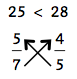﻿﻿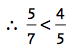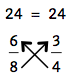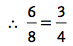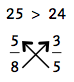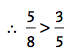The applet below ﻿helps you visualize fractions using number lines and circles so as to better understand how to compare fractions. Interact with the applet for a few minutes until you get a better understanding of the process of comparing fractions.
Below is a set of problems involving comparing fractions.